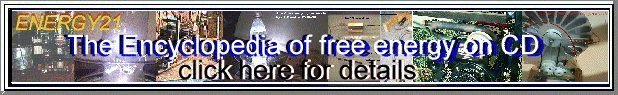### Basic Electrostatics

Electrostatics deals with charges, potentials, and the like where things aren't changing, i.e. they're static. Basic principles of electrostatics are used all the time in high voltage work for a lot of reasons. Popular high voltage generators like the Van de Graaf are based on electrostatic principles (even though a current is flowing). The burning question in a lot of high voltage work is whether the system will electrically breakdown as the voltage is raised. This is generally a question of quasi-static potential gradients which can be answered by simple electrostatics.

A collection of practical calculations based on the following theory.

### Coulomb's Law

The force on a charged point exerted by a second charge is proportional to the product of charges, and inversely proportional to the square of the distance between the charges, and acts either directly towards each other (opposite charges) or away from each other (same sign of charge).

F(vector) = k * q1 * q2 / r12^2 * direction(r12)

where

k = 1 / (4 * pi * epsilon) = 8.99E9 Newton Meter^2/Coulomb^2

where epsilon is the dielectric constant of the medium ( = 8.85E-12 for vacuum)

r12 is the scalar distance from point 1 to point 2

direction (r12) is a unit vector from point 1 to point 2

q1,q2 are charges on each point

#### Capacitance of two parallel plates

C = epsilon * Area / DistanceBetweenPlates

this neglects fringing effects, which for plates that are smaller than, say, 10 times the spacing, are pretty significant.

#### Capacitance of two concentric cylinders (e.g. coaxial cable)

C = 2 * pi * epsilon * length / ln( rOuter/rInner)

this assumes length >> r

#### Capacitance of two concentric spheres

C = 4 * pi * epsilon * rInner * rOuter/ (rOuter - rInner)

as rOuter goes to infinity, the fraction rOuter/(rOuter-rInner) goes to one, leading to the following handy equation:

#### Capacitance of isolated sphere

C = 4*pi*epsilon*radius = approx 111.2 pF/meter

this equation is derived from the equation for two concentric (nested) spheres, and letting the radius of the outer sphere go to infinity.

Copyright 1997, Jim Lux / 14 Sep 1997 / statics.htm / Back to HV Home / Back to home page / Mail to Jim